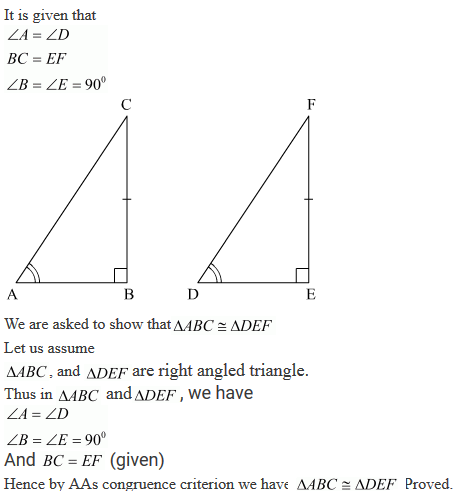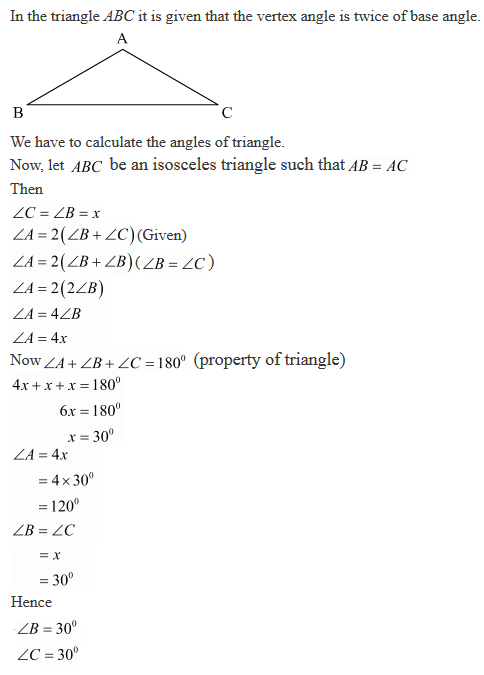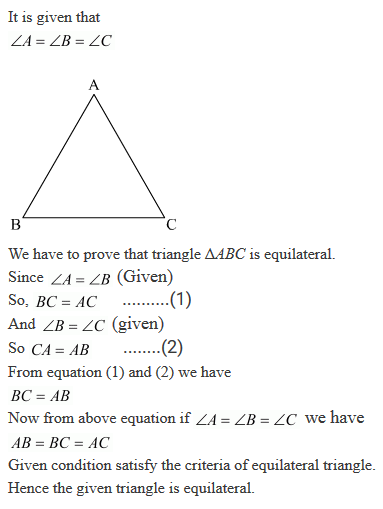#### Chapter 10 Congruent Triangles R.D. Sharma Solutions for Class 9th Exercise 10.3

Exercise 10.3

1. In two right triangles one side an acute angle of one are equal to the corresponding side and angle of the other. Prove that the triangles are congruent.

Solution2. If the bisector of the exterior vertical angle of a triangle be parallel to the base. Show that the triangle is isosceles.

Solution3. In an isosceles triangle, if the vertex angle is twice the sum of the base angles, calculate the angles of the triangle.

Solution4. PQR is a triangle in which PQ = PR and S is any point on the side PQ. Through S, a line is drawn parallel to QR and intersecting PR at T. Prove that PS = PT.

Solution5. In a ΔABC, it is given that AB = AC and the bisectors of ∠B and ∠C intersect at O. If M is a point on BO produced prove that ∠MOC = ∠ABC.

Solution6. P is a point on the bisector of an angle ∠ABC. If the line through P parallel to AB meets BC at Q, prove that triangle BPQ is isosceles.

Solution7. Prove that each angle of an equilateral triangle is 60°

Solution8. Angles A, B, C of a triangle ABC are equal to each other. Prove that ΔABC is equilateral.

Solution9. ABC is a triangle in which ∠B = 2∠C. D is a point on BC such that AD bisects ∠BAC and AB = CD. Prove that ∠BAC = 72°.

Solution

It is given that in ΔABC,
∠B = 2∠C
AB = CDTo prove: ∠BAC = 72

Now, let ∠C = y then ∠B = 2y (Given)
Since, AD is a bisector of ∠BAC
Let BP be the bisector of ∠ABC.
If we join PD we have,
In ΔBPC,
∠CBP = ∠BCP = y
So, BP = PC

In triangle ABP and DCP,
∠ABP = ∠DCP = y
AB = CD (Given)
BP = PC (Proved above)
So by SAS congruence criterion, we have ΔABP ≅ ΔADCP
⇒ ∠BAP = ∠CDP and AP = DP

∠CDP = 2x, and ∠ADP = ∠DAP = x (since ∠A = 2x)
In ΔABD,
we have ∠ADC = ∠ABD+ ∠BAC
= x+2x = 3x
So,
3x = x+2y
⇒ 2x = 2y
⇒ x= y
In ABC,
we have ∠A+ ∠B+ ∠C = 180°
⇒ 2x + 2y + y = 180°
⇒ 5x = 180°
⇒ x = 36°

Here,∠BAC = 2x = 2×36 = 72°

10. ABC is a right angled triangle in which ∠A = 90° and AB = AC. Find ∠B and ∠C.

Solution

It is given that,
∠A = 90°
AB = AC
We have to find ∠B and ∠C.
Since, AB = AC, therefore ∠B=∠C
Now, ∠A+ ∠B+ ∠C = 180° (property of triangle)
⇒ 90° + ∠B+ ∠B = 180° (Since ∠B= ∠C)
⇒ 90° +2∠B = 180°
⇒ 2∠B = 90°
⇒ ∠B = 90°/2
⇒ ∠B = 45°
Here, ⇒ ∠B = ∠C = 45°
Then, ∠A = 90°
Hence,
∠B = 45°
∠C = 45°